﻿ 电网谐波电流小波变换实时检测研究

# 电网谐波电流小波变换实时检测研究Real-Time Detection of Harmonic Current Wavelet Transform in Power Grid

Abstract: In the harmonic control and reactive power compensation of the power grid, real-time detection and analysis of harmonics and reactive currents in the power grid are needed to suppress and compensate the reactive power in the harmonic current in the power grid. In this paper, the ap-plication of wavelet transform algorithm in power grid harmonic current detection is studied. The algorithm uses the mallat decomposition algorithm to obtain the wavelet decomposition coefficients of each frequency band, and then reconstructs the wavelet coefficients obtained by decomposition separately, so that the base can be separated. This allows the fundamental current and the harmonic components to be separated. The simulation and experimental results show that the proposed method can separate the fundamental current and each harmonic well, and can meet the requirements of real-time detection of harmonic current.

1. 引言

• 基于傅立叶变换的谐波检测方法；

• 基于瞬时无功功率理论的谐波检测方法；

• 基于人工神经网络的谐波分析和检测方法；

• 基于小波变换的谐波分析与检测方法。

2. Mallat算法

$\begin{array}{l}\varphi \left(t\right)=\sqrt{2}\underset{n=-\infty }{\overset{+\infty }{\sum }}h\left(n\right)\varphi \left(2t-n\right)\\ \phi \left(t\right)=\sqrt{2}\underset{n=-\infty }{\overset{+\infty }{\sum }}g\left(n\right)\phi \left(2t-n\right)\end{array}$ (1)

${c}_{j,k}=\underset{m=-\infty }{\overset{+\infty }{\sum }}h\left(m-2k\right){c}_{j-1,m}$ (2)

${d}_{j,k}=\underset{m=-\infty }{\overset{+\infty }{\sum }}g\left(m-2k\right){c}_{j-1,m}$ (3)

Mallat算法是小波变换的快速算法，Mallat算法为小波变换在实际应用分析中奠定了基础，接下来我们对Mallat算法进行研究。

$x\left(t\right)=\underset{k=-\infty }{\overset{+\infty }{\sum }}{c}_{j-1,k}{2}^{\left(-j+1\right)/2}\varphi \left({2}^{-j+1}t-k\right)$ (4)

$x\left(t\right)=\underset{k=-\infty }{\overset{+\infty }{\sum }}{c}_{j,k}{2}^{-j/2}\varphi \left({2}^{-j}t-k\right)+\underset{k=-\infty }{\overset{+\infty }{\sum }}{d}_{j,k}{2}^{-j/2}\varphi \left({2}^{-j}t-k\right)$ (5)

$x\left(t\right)=\underset{j=-\infty }{\overset{j}{\sum }}\underset{k=-\infty }{\overset{+\infty }{\sum }}{d}_{j,k}\left(t\right){\phi }_{j,k}\left(t\right)+\underset{k=-\infty }{\overset{+\infty }{\sum }}{c}_{j,k}\left(t\right){\varphi }_{j,k}\left(t\right)$ (6)

${c}_{j-1,m}=\underset{k=-\infty }{\overset{+\infty }{\sum }}{c}_{j,k}\left(t\right)h\left(m-2k\right)+\underset{k=-\infty }{\overset{+\infty }{\sum }}{d}_{j,k}\left(t\right)g\left(m-2k\right)$ (7)

3. 基于小波变换谐波电流检测

3.1. 电网电流的小波分解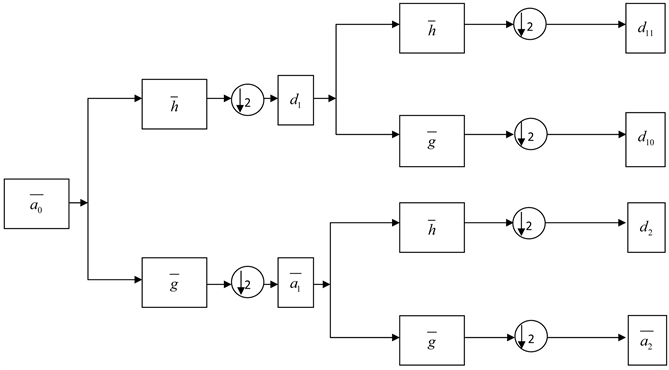Figure 1. Schematic diagram of signal n-time decomposition

3.2. 基波电流的重构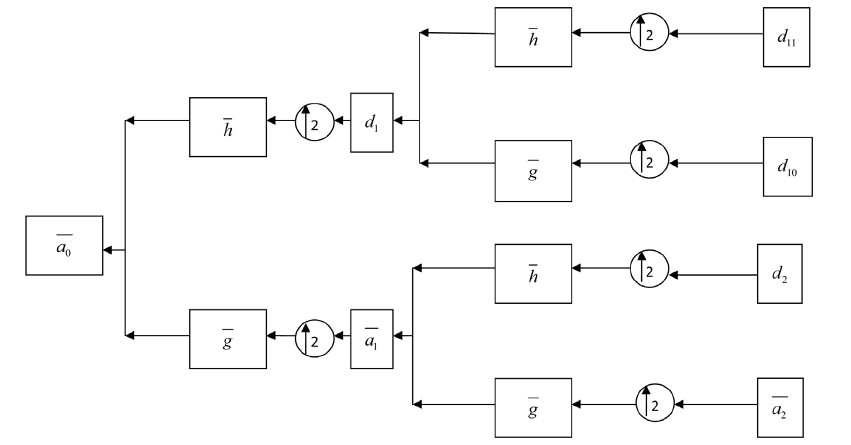Figure 2. Schematic diagram of signal reconstruction

3.3. 仿真分析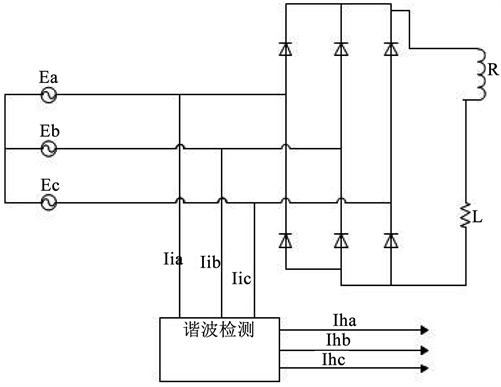Figure 3. Harmonic detection circuit structure

l 确定小波空间。将采样信号谐波电流 ${\text{i}}_{\text{la}}$ 根据谐波成分进行小波分解。基波电流系数位于第5层低频概貌中。

l 进行5层小波分解，将 ${\text{i}}_{\text{la}}$ 作为 ${V}_{0}$ 空间的低频 ${C}_{0,k}$ ，对信号进行5次2抽取，保证数据总长度与输入信号长度相等。得到不同频率段的小波变换系数。

l 将基波电流小波系数 ${C}_{5,k}$ 单独重构，得到基波电流 ${C}_{5}$

l 在电网电流中减去得到的基波电流就可以得到谐波电流。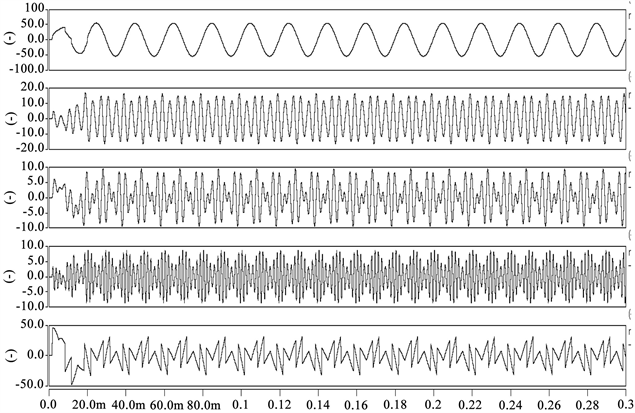Figure 4. Detected fundamental current, separated harmonic currents and harmonic current waveforms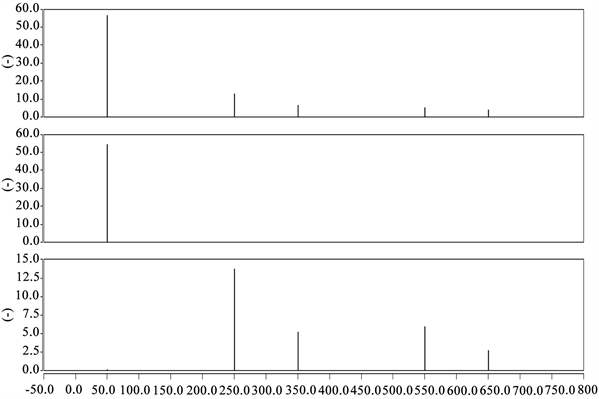Figure 5. Grid current, fundamental current and separated harmonic current spectrum

4. 全文总结

 王兆安. 谐波抑制和无功功率补偿[M]. 北京: 机械工业出版社, 1998: 1-2.

 于军. 基于DSP+ARM的电能质量分析仪的研究与设计[D]: [硕士学位论文]. 上海: 东华大学, 2016.

 林华伦. 基于傅里叶和小波变换的电网谐波检测方法研究[D]: [硕士学位论文]. 南宁: 广西大学, 2013

 陈欢, 何怡刚, 肖建平, 刘茂旭, 王东楼. 基于CWT和DWT相结合的谐波检测[J]. 电力系统保护与控制, 2015, 43(20): 71-75.

 彭玉华. 小波变换与工程应用[M]. 北京: 科学出版社, 2003: 75-77.

 雷汝海, 郝震. 基于小波变换Mallat算法的电网谐波检测方法[J]. 工矿自动化, 2014, 40(12): 65-69.

 杨福生. 小波变换的工程分析与应用[M]. 北京: 科学出版社, 2000: 121-123.

 范芳芳. 基于神经网络与小波分析的电力系统谐波检测研究[D]: [硕士学位论文]. 南昌: 南昌大学, 2017.

 郭涛. 基于小波变换和加窗FFT的谐波检测的研究[J]. 科学经济导刊, 2018, 26(11): 1-2.

 吴勇. 有源电力滤波器若干关键技术研究[D]: [博士学位论文]. 武汉: 华中科技大学, 2007．

 唐忠. 焦婷. 基于小波包变换电力系统谐波分析[J]. 电测与仪表, 2016, 53(18): 57-62.

Top